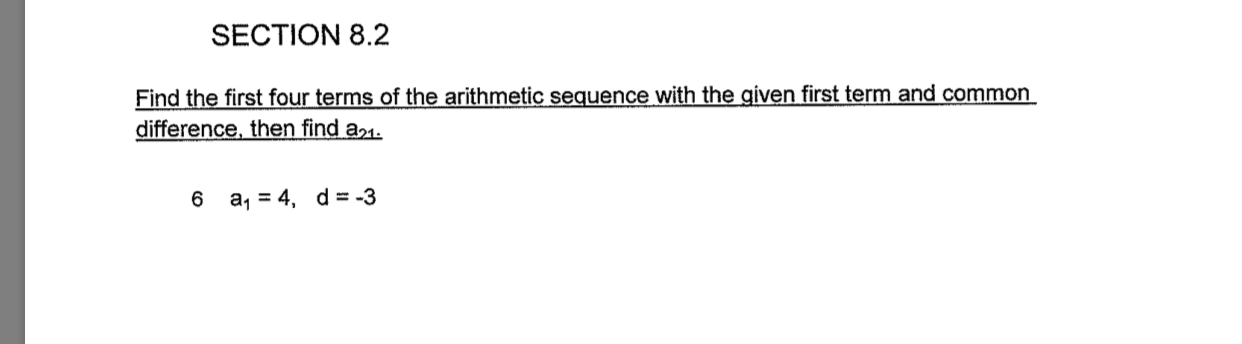SECTION 8.2Find the first four terms of the arithmetic sequence with the given first term and commondifference, then find a16 a1 4, d -3

Questionhelp_outlineImage TranscriptioncloseSECTION 8.2 Find the first four terms of the arithmetic sequence with the given first term and common difference, then find a1 6 a1 4, d -3 fullscreen
Step 1

The terms of an arithmetic sequence can be obtained by adding common difference to its preceding term.

Step 2

The twenty first term can be obtained by substituting the values of a1=4, d=-3 and...

Want to see the full answer?

See Solution

Want to see this answer and more?

Our solutions are written by experts, many with advanced degrees, and available 24/7

See Solution
Tagged in

Sequence and Series